## Number Notation and Place Values, Comparing and Ordering, Number Patterns, Rounding Off or Approximation and Estimation

Practice Unlimited Questions

#### How to round off a number to the nearest ten:

1. Study the digit in the ones place.
1. If it is equal to or more than 5, add 1 to the digit in the tens place (i.e. round up)
2. If it is less than 5, keep the digit in the tens place unchanged (i.e. round down)

2. Now, change the digit in the ones place to 0.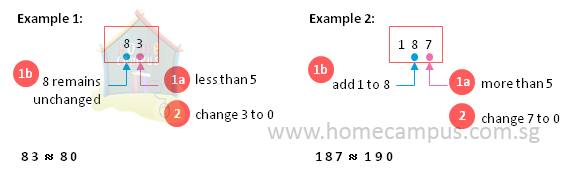#### How to round off a number to the nearest hundred:

1. Study the digit in the tens place.
1. If it is equal to or more than 5, add 1 to the digit in the hundreds place (i.e. round up)
2. If it is less than 5, keep the digit in the hundreds place unchanged (i.e. round down)

2. Now, change the digit in the tens and ones places to 0s.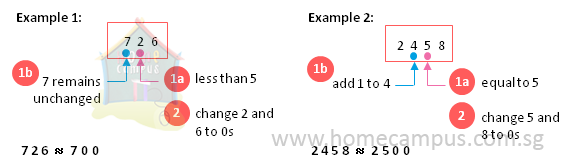#### 1. Kelly has \$25 439 in her savings account. What is this amount in words?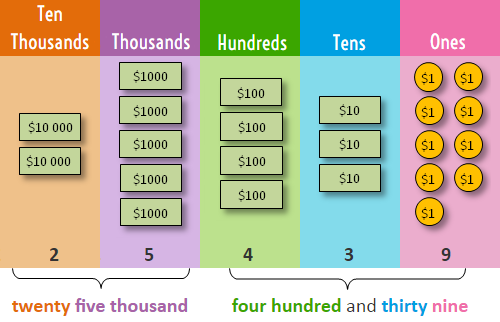So, in words, Kelly has twenty five thousand, four hundred and thirty nine dollars in her savings account.

#### 2. Find the place and value of each digit in the number 25 439.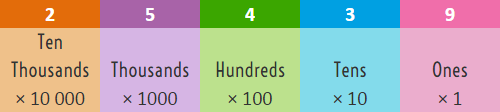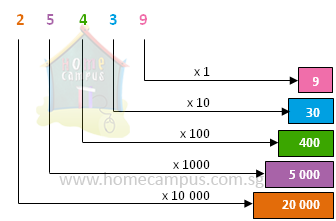In 25 439,
• the digit 9 is in the ones place, its value is 9 × 1 or 9
• the digit 3 is in the tens place, its value is 3 × 10 or 30
• the digit 4 is in the hundreds place, its value is 4 × 100 or 400
• the digit 5 is in the thousands place, its value is 5 × 1000 or 5000
• the digit 2 is in the ten thousands place, its value is 2 × 10 000 or 20 000

#### 3. How do you write sixty thousand and twenty three in figures?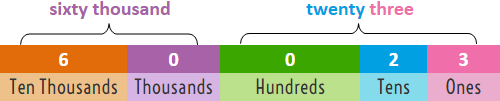sixty thousand  =  60  ×  1000  =  60 000
twenty three  =  23  ×  1  =  23

So,
sixty thousand and twenty three  =  60 000  +  23  =  60 023

In figures, sixty thousand and twenty three is 60 023.

#### 4. How many hundreds are there in 36 000?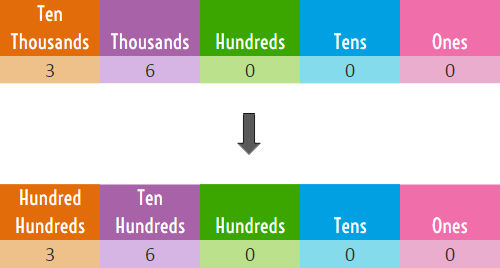36 000
=  360 00
=  360 hundreds

There are 360 hundreds in 36 000.
There are three hundred and sixty hundreds in thirty six thousand.

#### 5. Compare the numbers 37 261, 38 412 and 37 265. a. Which number is the smallest? b. Which number is the biggest? c. Arrange the numbers in order, beginning with the smallest.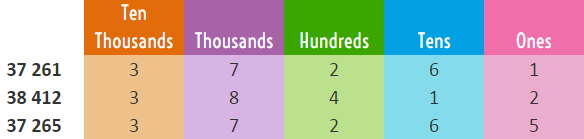Compare the numbers digit by digit starting with the digit in the highest place value.
a.  37 261 is the smallest number
b.  38 412 is the biggest number
c.  37 261, 37 265, 38 412

#### 6. Complete the number patterns below. a.  600,   725,   _______,   975,   ________,   1225 b.  45 554,   46 554,   46 454,   47 454,   47 354,   ________,   ________,   49 254

a.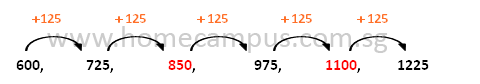Rule: Add 125 to get the next number.

b.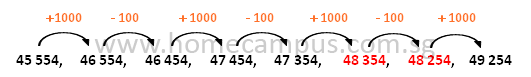Rule: Add 1000 and subtract 100 alternately to continue the number pattern.

#### 7.  How do you round off a number to the nearest ten?

Rounding off or approximation means to simplify numbers so they are easy to use and remember.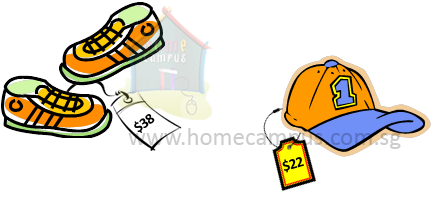a. Joyce bought a pair of shoes for \$38.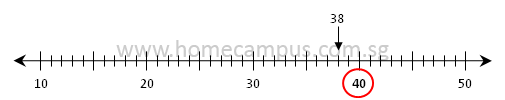\$38 is between 30 and 40.
It is nearer to 40 than to 30.

\$38  ≈  \$40
Joyce bought the shoes for about \$40.

We round up 38 to the nearest ten to get 40.

We use to denote approximately equal to.
b. Jarrel bought a cap for \$22.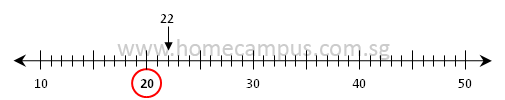\$22 is between 20 and 30.
It is nearer to 20 than to 30.

\$22 ≈ \$20
Jarrel bought the cap for about \$20.

We round down 22 to the nearest ten to get 20.

#### 8.  How do you round off a number to the nearest hundred?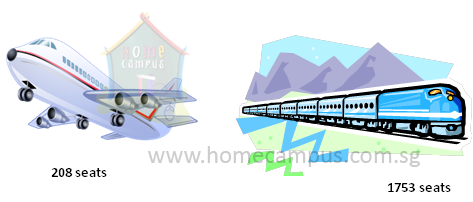a.  How many passengers can the aeroplane hold?
b.  How many passengers can the train hold?

a. The aeroplane has 208 seats.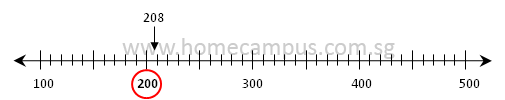208 is between 200 and 300.
It is nearer to 200 than to 300.

208 ≈ 200
The aeroplane can hold about 200 passengers.

We round down 208 to the nearest hundred to get 200.
b. The train has 1753 seats.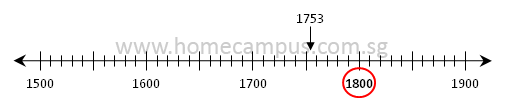1753 is between 1700 and 1800.
It is nearer to 1800 than to 1700.

1753 ≈ 1800
The train can hold about 1800 passengers.

We round up 1753 to the nearest hundred to get 1800.

#### 9. Estimate the value of: a.  412  +  37 b.  2187  −  213 c.  586  ×  4 d.  641  ÷  7

a.  412  +  37
≈ 410  +  40
= 450

412 is 410 when rounded off to the nearest ten.

37 is 40 when rounded off to the nearest ten.
b.  2187  −  213
≈ 2200  −  200
= 2000

2187 is 2200 when rounded off to the nearest hundred.

213 is 200 when rounded off to the nearest hundred.
c.  586  ×  4
≈ 600  ×  4
= 2400

586 is 600 when rounded off to nearest hundred.
d.  651  ÷  7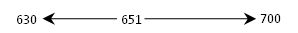651  ÷  7
≈ 630  ÷  7
= 90
Think of multiples of 7.
..., 56, 63, 70, 77, ...
..., 560, 630, 700, 770, ...
651 is nearer to 630 than to 700.